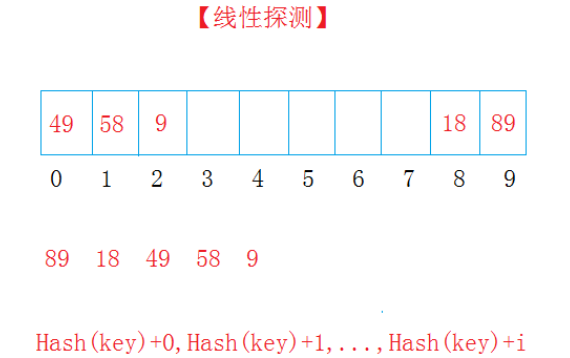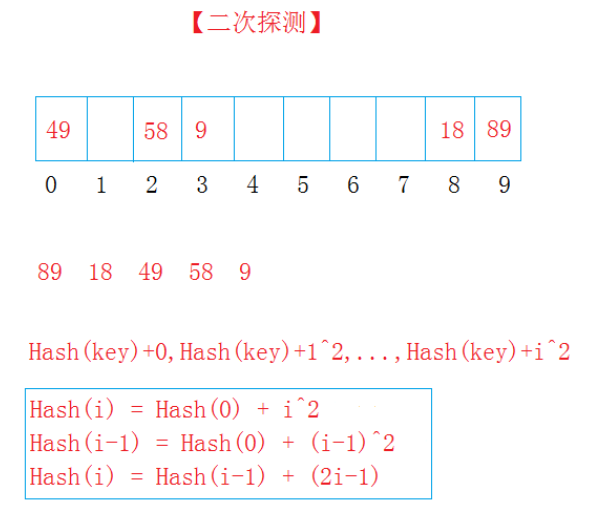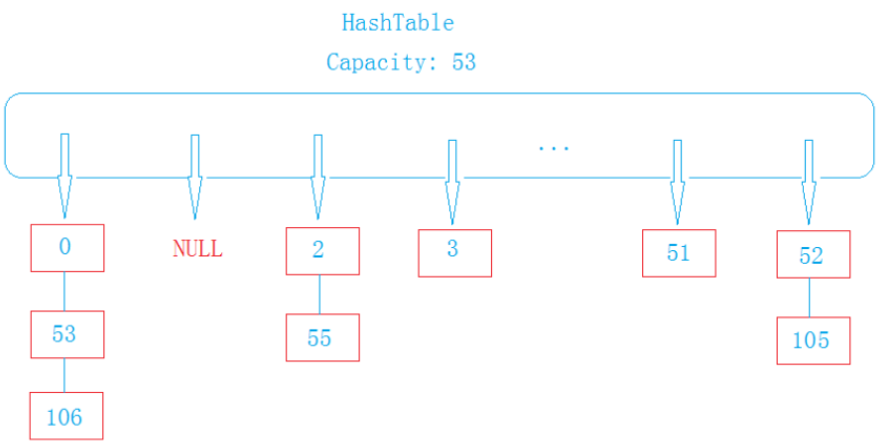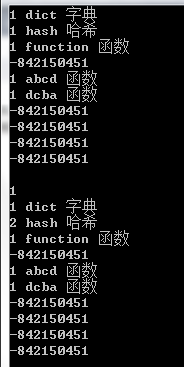• 构造哈希表之二次探测法

万次阅读 多人点赞 2016-06-08 01:19:00
HashTable-散列表/哈希表 是根据关键字（key）而直接访问在内存存储位置的数据结构。 它通过一个关键值的函数将所需的数据映射到表中的位置来访问数据，这个映射函数叫做散列（哈希）函数，存放记录的数组叫做散列表...

HashTable-散列表/哈希表

是根据关键字（key）而直接访问在内存存储位置的数据结构。

它通过一个关键值的函数将所需的数据映射到表中的位置来访问数据，这个映射函数叫做散列（哈希）函数，存放记录的数组叫做散列表。

构造哈希表的几种方法
1.直接定址法（取关键字的某个线性函数为哈希地址）
2.除留余数法（取关键值被某个不大于散列表长m的数p除后的所得的余数为散列地址）
3.平方取中法
4.折叠法
5.随机数法
6.数学分析法
常用方法是 直接定址法除留余数法

哈希冲突/哈希碰撞
不同的Key值经过哈希函数Hash(Key)处理以后可能产生相同的值哈希地址，我们称这种情况为哈希冲突。任意的散列函数都不能避免产生冲突。

处理哈希碰撞的方法
若key1,key2,key3产生哈希冲突(key1,key2,key3值不相同，映射的哈希地址同为key)，用以下方法确定它们的地址

1.闭散列法
1）线性探测
若当前key与原来key产生相同的哈希地址，则当前key存在该地址之后没有存任何元素的地址中
key1：hash(key)+0
key2：hash(key)+1
key3：hash(key)+2
例如：2）二次探测
若当前key与原来key产生相同的哈希地址，则当前key存在该地址后偏移量为（1,2,3...）的二次方地址处
key1：hash(key)+0
key2：hash(key)+1^2
key3：hash(key)+2^2
例如：2.开链法（哈希桶）
哈希表中保存包含每个key值的节点，每个节点有一个_next的指针，指向产生哈希冲突的key的节点
例如：具体实现方式请看下一篇博客，博客链接： http://blog.csdn.net/xyzbaihaiping/article/details/51610944

构建哈希表（二次探测法）
支持key值为字符串
<pre name="code" class="cpp">//HashTable.h
#pragma once
#include<iostream>
#include <string>
using namespace std;
enum State
{
EMPTY,//空
EXITS,//存在
DELETE//已删除
};

template<class K, class V>
struct HashTableNode
{
K _key;
V _value;
};

template<class K>
struct _HashFunc
{
size_t operator()(const K& key,const size_t& capacity)//哈希函数，仿函数
{
return key / capacity;
}

};
template<>
struct _HashFunc<string>//模板特化
{
private:
unsigned int _BKDRHash(const char *str)//key为字符串时哈希函数
{
unsigned int seed = 131; // 31 131 1313 13131 131313 etc..
unsigned int hash = 0;

while (*str)
{
hash = hash * seed + (*str++);
}
return (hash & 0x7FFFFFFF);
}
public:
size_t operator()(const string& key,const size_t& capacity)//仿函数
{

return _BKDRHash(key.c_str()) % capacity;
}

};
template<class K, class V,class HashFunc=_HashFunc<K>>
class HashTable
{

typedef HashTableNode<K, V> Node;
public:
HashTable(size_t capacity = 10)
:_tables(new Node[capacity])
, _states(new State[capacity])
, _size(0)
, _capacity(capacity)
{}
~HashTable()
{
if (_tables != NULL)
{
delete[] _tables;
delete[] _states;
}

}
HashTable(const HashTable<K, V>& ht)
{
HashTable<K, V> tmp(ht._capacity);
for (size_t i = 0; i < ht._capacity; i++)
{
tmp.Insert(ht._tables[i]._key, ht._tables[i]._value);
}
this->Swap(tmp);
}
HashTable& operator=(HashTable<K, V> ht)
{
this->Swap();
return *this;
}
bool Insert(const K& key, const V& value)
{
_CheckCapacity();
size_t index = HashFunc()(key, _capacity);
size_t i = 1;
while (_states[index] == EXITS)//二次探测
{
if (_tables[index]._key == key)
{
return false;
}
index = index + 2 * i - 1;
index %= _capacity;
++i;
}
_tables[index]._key = key;
_tables[index]._value = value;
_states[index] = EXITS;
++_size;
return true;
}

bool Find(const K& key)
{
size_t index = HashFunc()(key, _capacity);
size_t start = index;
size_t i = 1;
while (_states[index] != EMPTY)//根据二次探测法查找
{
if (_tables[index]._key == key)
{
if (_states[index] != DELETE)
return true;
else
return false;
}
index = index + 2 * i - 1;
index %= _capacity;
if (start == index)
return false;
}
return false;
}
bool Remove(const K& key)
{
size_t index = HashFunc()(key, _capacity);
size_t start = index;
size_t i = 1;
while (_states[index] != EMPTY)//根据二次探测法删除
{
if (_tables[index]._key == key)
{
if (_states[index] != DELETE)
{
_states[index] = DELETE;
_size--;
return true;
}
else
return false;
}
index = index + 2 * i - 1;
index %= _capacity;
if (start == index)
return false;
}
return false;

}
void Print()
{
for (size_t i = 0; i < _capacity; i++)
{
//printf("%d-[%s:%s] \n", _states[i], _tables[i]._key, _tables[i]._value);
cout << _states[i] << " " << _tables[i]._key << " " << _tables[i]._value<<endl;
}
}
private:
void Swap(HashTable<K, V>& tmp)
{
swap(_tables, tmp._tables);
swap(_states, tmp._states);
swap(_size, tmp._size);
swap(_capacity, tmp._capacity);
}
void _CheckCapacity()//增容
{
if (_size * 10 / _capacity == 6)
{
HashTable<K, V> tmp(_capacity * 2);
for (size_t i = 0; i < _capacity; i++)
{
if (_states[i] == EXITS)
tmp.Insert(_tables[i]._key, _tables[i]._value);
}
this->Swap(tmp);
}
}

private:
Node* _tables;//哈希表
State* _states;//状态表
size_t _size;
size_t _capacity;
};

</pre><pre code_snippet_id="1711228" snippet_file_name="blog_20160608_3_3809584" name="code" class="cpp">//test.cpp
#include<iostream>
#include "HashTable.h"
void testInt()
{
HashTable<int, int> table(10);
table.Insert(89, 89);
table.Insert(18, 18);
table.Insert(49, 49);
table.Insert(58, 58);
table.Insert(9, 9);
//table.Insert(45, 45);
//table.Insert(2, 2);
table.Print();
HashTable<int, int> table1(table);
table1.Print();
bool ret = table.Find(9);
cout << endl << ret << endl;
table.Remove(9);
table.Print();

}
void TestString()
{
HashTable<string, string> table(10);
table.Insert("dict", "字典");
table.Insert("hash", "哈希");
table.Insert("function", "函数");
table.Insert("abcd", "函数");
table.Insert("dcba", "函数");

table.Print();
bool ret = table.Find("function");
cout << endl << ret << endl;
table.Remove("hash");
table.Print();
}
int main()
{
//testInt();
TestString();
getchar();
return 0;
}

测试结果：展开全文闭散列法
• #include<iostream> #include<time.h> #include<string> #include<math.h>...#define m 15//哈希表的表长 ...//HT是哈希表，HC是统计每个元素的插入时候的查找次数。并且初始化。 int H

#include<iostream>
#include<time.h>
#include<string>
#include<math.h>
#include<stdio.h>
#define m 15//哈希表的表长
#define NullKey 0 //单元为空的标记
using namespace std;
int HT[m] = {}, HC[m] = {};//HT是哈希表，HC是统计每个元素的插入时候的查找次数。并且初始化。
int H(int key) {//哈希函数
return key % 13;
}
//线性探测，既可以找到空的地方，没空的地方也可以找到是哈希表之前是否有这个值，查找中，如果第一次没找到，则调用这个函数，继续往下查找。
int Linedetece(int HT[], int H0, int key, int& cnt) {//线性探测法  参数：哈希表，之前哈希函数计算出来的下标值，关键字，查找次数
int HI;
for (int i = 1; i < m; ++i) {
cnt++;
HI = (H0 + i) & m;//按照线性探测法计算下一个哈希地址HI
if (HT[HI] == NullKey) {
return HI;
}
else if(HT[HI]==key)//如果找到和这个关键字重复的值，返回该下标 ,,若单元HI中元素的关键字为Key
//查找会用到，插入的话不会用到这一步，因为如果插入有重复的会插入失败；
{
return HI;
}
}
return -1;
}
//二次探测，既可以找到空的地方，没空的地方也可以找到是哈希表之前是否有这个值，查找中，如果第一次没找到，则调用这个函数，继续往下查找。
int Seconddetect(int HT[], int H0, int key, int& cnt) {//二次探测法。
int HI;
for (int i = 1; i <= m/2; ++i) {
int i1 = i * i;//右边
int i2 = -i1;//左边
cnt++;
HI = (H0 + i1) % m;//按照线性探测法计算下一个哈希地址
if (HT[HI] == NullKey) {//若单元HI为空，则所查元素不存在。
return HI;
}
else if (HT[HI] == key) {//若单元HI中元素的关键字为Key
return HI;
}
cnt++;//因为一个下标要查左右两次，所以需要两次++;
HI = (H0 + i2) % m;
if (HI < 0) HI += m;//如果超出左边界。右边界不可能超出，左边界可能变为负数
if (HT[HI] == NullKey) {
return HI;
}
if (HT[HI] == key) {
return HI;
}
}
return -1;
}
//查找，第一种情况：第一次的位置为空， 则插入  第二种情况，第一次位置不为空，被占据，按线性往后找空的插入。都没找到返回0；
bool InsertHash(int HT[], int key) {
int H0 = H(key);
int HI = -1, cnt = 1;
if (HT[H0] == NullKey) {
HC[H0] = 1;//统计查找次数
HT[H0] = key;//若单元H0为空，放入
return true;
}
else {
HI = Linedetece(HT, H0, key, cnt);//找到后面空的下标，或者和自己数字相等的下标，相等的下标情况会在下面的if去掉。
//HI = Seconddetect(HT, H0, key, cnt);线性查找
if ((HI != -1) && (HT[HI] == NullKey)) {//哈希表里面有空值，如果没有空值，则失败，
//如果哈希表没满或者该元素没有插入  //因为上面的探测函数有可能找到key值，也会将其下标返回。哈希表不能有重复的值。

HC[HI] = cnt;
HT[HI] = key;//若单元H0为空，放入
return true;
}
}
return 0;
}
void print(int HT[])
{
for (int i = 0; i < m; i++) {
cout << HT[i] << "\t";
}
cout << endl;
}
//查找，第一种情况，查是不是空，第二种情况，查第一次有没有找到，第三种情况，查哈希表其他位置有没有，第四种情况 返回失败。
int SerachHasn(int HT[], int key)
{
//在哈希表HT中查找关键字为key的元素，若查找成功，则返回哈希表的单元序号。
int H0 = H(key);//根据哈希函数key计算哈希地址。
int HI, cnt = 1;
if (HT[H0] == NullKey) {//若单元H0为空，则所查元素不存在
return -1;
}
else if(HT[H0]==key){//若单元HO中元素的关键字为key，则查找成功。
cout << "查找成功，比较次数：" << cnt << endl;
return 0;
}
else {//第一次查找不为空，也没找到（是因为之前的元素占据了这个元素应该初始所在位置）
HI = Linedetece(HT, H0, key, cnt);
if (HT[HI] == key) {//若单元HO中元素的关键字为key，则查找成功。
cout << "查找成功，比较次数：" << cnt << endl;
return HI;
}
else
{
return -1;//若单元H0为空，则所查元素不存在
}
}
}
int main()
{
int x;

//memset(HT, 0, sizeof(HT));//将哈希表HT的sizeof（HT）个字节用0替换。
//memset(HT, 0, sizeof(HC));
print(HT);
cout << "输入12个关键字，存入哈希表中" << endl;
for (int i = 0; i < 12; i++) {
cin >> x;
if (!InsertHash(HT, x)) {
cout << "创建哈希表失败" << endl;
return 0;
}
}
cout << "输出哈希表" << endl;
print(HT);//输出哈希表
print(HC);//输出记录每个元素查找次数的数组。
cout << "输入要查找的关键字" << endl;
cin >> x;
int result = SerachHasn(HT, x);
if (result == -1) {
cout << "没找到";
}
else
{
cout << "在第" << result + 1 << "的位置找到" << endl;
}
return 0;

}

展开全文• 这是数据结构课程作业，用二次探测再散列法解决冲突建立哈希表并查找 从键盘读入 待查找 的权重数值，以除留余数法为哈希函数，二次探测再散列法解决冲突建立哈希表，基于哈希算法从数组中查找相应的记录，计算相应...
• 假设数组hashTable的长度是mSize，用平方探测法插入数字num，每次的位置pos = (num + i * i) % mSize，i的范围是[0, mSize)。 bool flag = false; //未插入 for(int i = 0; i < mSize; i++){ int pos = (num + i...

假设数组hashTable的长度是mSize，用哈希算法的方式插入数字num，同时使用二次探测法（正向）来解决冲突。则每次的位置pos = (num + i * i) % mSize，i的范围是[0, mSize)。

bool flag = false; //未插入
for(int i = 0; i < mSize; i++){
int pos = (num + i * i) % mSize;
if(hashTable[pos] == 0){
hashTable[pos] = num;
flag = true; //已插入
break;
}
}
if(flag == false){
printf("%d can't be inserted.", num);
}

展开全文• DS哈希查找—二次探测再散列

万次阅读 多人点赞 2018-12-04 17:23:03
输入表长（大于、等于11），输入关键字集合，用二次探测再散列构建哈希表，并查找给定关键字。 输入 测试次数t 每组测试数据格式如下： 哈希表长m、关键字个数n n个关键字 查找次数k k个待查关键字 输出 对...

题目描述

定义哈希函数为H(key) = key%11。输入表长（大于、等于11），输入关键字集合，用二次探测再散列构建哈希表，并查找给定关键字。

输入

测试次数t

每组测试数据格式如下：

哈希表长m、关键字个数n

n个关键字

查找次数k

k个待查关键字

输出

对每组测试数据，输出以下信息：

构造的哈希表信息，数组中没有关键字的位置输出NULL

k个待查关键字，分别输出：

010—不成功，1—成功）、比较次数、查找成功的位置（从1开始）

样例输入

1

12 10

22 19 21 8 9 30 33 4 41 13

4

22

15

30

41

样例输出

22 9 13 NULL 4 41 NULL 30 19 8 21 33

1 1 1

0 3

1 3 8

1 6 6

思路：

取key的方式：先取余，若不冲突则直接存，若冲突则加上偏移量(1²,-1²,2²,-2²......)，然后在长为m的hash表中循环滚动，最后确定key

key第一次取value%11

如果位置冲突，key取：value % 11 + 1²，如果key超过hash表的长度m，key取key-m，如果key的值为负，key取key+m

如果位置冲突，key取：value % 11 + （-1²），如果key超过hash表的长度m，key取key-m，如果key的值为负，key取key+m

如果位置冲突，key取：value % 11 + （2²），如果key超过hash表的长度m，key取key-m，如果key的值为负，key取key+m

如果位置冲突，key取：value % 11 + （-2²），如果key超过hash表的长度m，key取key-m，如果key的值为负，key取key+m

以此类推下去取key

code:

#include <iostream>
using namespace std;

void test()
{
int m,n;
cin>>m>>n;
int *hashh;
hashh = new int [m];
for(int i=0;i<m;i++)
hashh[i]=-100000;
int value,key,flag,temp_key,d,symb,num;
for(int i=0;i<n;i++)                //建hash表
{
cin>>value;
key=value%11;
temp_key=key;
d=1;                            //偏移量
symb=1;                         //符号
num=0;                          //hash次数
while(1)
{
if(hashh[temp_key]==-100000)
{
hashh[temp_key]=value;
break;
}
else
{                                    //滚动取key
num++;
temp_key=key+symb*(d*d);    //更新key
symb*=-1;                //更新符号
if(num%2==0)            //每2次需要更新1次偏移量
d++;
if(temp_key>m)            //key超过hash表长度
temp_key -= m;
else if(temp_key <0)      //key的值为负数
temp_key += m;
}
}
}
for(int i=0;i<m;i++)
{
if(hashh[i] == -100000)
cout<<"NULL";
else
cout<<hashh[i];
if(i != m-1)
cout<<' ';
}
cout<<endl;

int search_num,search_time;
cin>>search_num;
for(int i=0;i<search_num;i++)            //查找
{
search_time=0;
cin>>value;
key=value%11;
temp_key=key;
d=1;
symb=1;
flag=0;
num=0;
while(1)
{
search_time++;
if(hashh[temp_key]==value)
{
flag=1;
cout<<1<<' '<<search_time<<' '<<temp_key+1<<endl;
break;
}
else
{                                    //和建表一样的更新方法
if(hashh[temp_key]==-100000)
break;
num++;
temp_key=key+symb*(d*d);
symb*=-1;
if(num%2==0)
d++;
if(temp_key>m)
temp_key -= m;
else if(temp_key <0)
temp_key += m;
if(num>m/2)
break;
}
}
if(flag==0)
cout<<0<<' '<<search_time<<endl;
}
delete[] hashh;
}
int main()
{
int t;
cin >> t;
while (t--)
{
test();
}

return 0;
}

展开全文再散列
• 哈希二次探测公式： h i = ( h a s h ( k e y ) + i ∗ i ) % S i z e , 0 < = i < = S i z e − 1 h_i = (hash(key) + i *i) \% Size, 0 #include #include #include #include using namespace...pat hash
• 哈希表线性探测&二次探测

千次阅读 2017-06-09 11:00:36
在代码中实现了哈希表中任意类型都可以存放，即哈希函数要可扩展以及哈希表动态增容的功能。 贴上代码： #include #include using namespace std; template//特化 class _HashFun { public: size_t operator...数据结构 哈希表 二次探测
• HashTable的简单介绍HashTable是根据关键字直接访问在内存存储的数据结构。 HashTable叫哈希表或者散列表。 它通过一个关键值的函数将所需的数据...给定一字符串“abckhdgubhkacdggk”找出第一只出现一的字符。hashtable 散列函数 数据结构 哈希冲突
• 构造哈希表以及二次探测法

千次阅读 2018-09-16 19:53:39
构造哈希表（散列表）以及二次探测法 今天做笔试题时，遇到一道构造哈希表的题，hash函数是 k%11 ，然后一个数组记不清了，然后就是问二次探测法进行，问下面那个是正确，懵逼啊，没做过，不知道，乱选直接下...Java 二次探测法
• 知道如下两个公式： Hash=key%size ...id就是要将X插入到哈希表里的下标值 第一行是已经插入的一个序列 第行是要插入的序列，判断会不会冲突 The task of this problem is simple: insert a sequenc...
• 二次探测是避免哈希冲突的一种常见手段，思想是：插入：找到哈希位置（serch）->如果不冲突就插入，冲突就进行第一次探测第1次探测：哈希位置变为原有哈希位置加上1*1的偏移->进行插入........第i次探测：哈希...
• 1078 Hashing (25 分) 题目传送门：1078 Hashing (25 分) ...在哈希时如果冲突，则二次探测再散列。刚开始没注意这一句:Quadratic probing (with positive increments only) is used to solve the...哈希表 二次探测再散列
• //二次探测法 index = (index + iCount*iCount) % Hash->capacity; iCount++; } Hash->arr[index].key = key; Hash->arr[index].state = EXISTED; return 1; } //扩容操作 //如果负载因子超标，则...
• 哈希表又称散列表。 哈希表存储的基本思想是：以数据表中的每个记录的关键字 k为自变量，通过一种函数H(k)计算出函数值。把这个值解释为一块连续存储空间（即数组空间）的单元地址（即下标），将该记录存储到这个...
• 问题描述从空表开始，将输入元素按照输入顺序逐个插入一个哈希表，以生成哈希表...=m)，用二次探测再散列法处理冲突，即探测序列为Hi=(Hash(key)+di) mod m，其中增量序列为di = 12, -12, 22, -22,32,-32…, k2, -k2...
• 理想的搜索方法是可以不经过任何比较，一直接从表中得到要搜索的元素。如果构造一种存储结构，使元素的存储位置与它的关键码之间建立一个确定的对应函数关系Hash()，那么每个元素关键码与结构中的一个唯一的存储.....
• 输入表长（大于、等于11），输入关键字集合，用二次探测再散列构建哈希表，并查找给定关键字。 输入 测试次数t 每组测试数据格式如下： 哈希表长m、关键字个数n n个关键字 查找次数k k个待查关键字 输出 对每组测试...数据结构 算法 hash
• 二次探测：若当前key与原来key产生相同的哈希地址，则当前key存在该地址后偏移量为（1,2,3…）的二次方地址处 key1：hash(key)+0 key2：hash(key)+1^2 key3：hash(key)+2^2 ··· 二次探测法中会每一个空间采用一个...哈希
• 问题 B: DS哈希查找—二次探测再散列(关键字互不相同） 时间限制: 1 Sec 内存限制: 128 MB 提交: 123 解决: 57 [提交][状态][讨论版] 题目描述 定义哈希函数为H(key) = key%11。输入表长（大于、等于11），输入...数据结构 算法 哈希
• 因此在查找一个数据时，必须经过关键码的多比较，搜索效率取决于比较次数。而一个理想的搜索是不经过比较，直接拿出数据，建立关键码和存储位置的关系。 哈希冲突的产生与解决 不同的关键码通过相同的哈希...
• //偶数冲突向后查找 NewPos = CurrentPos - ( CrashNum / 2 ) * ( CrashNum / 2 ) ; while ( NewPos < 0 ) //等于也不行 { NewPos = NewPos + h - > TableSize...
• 哈希+二次探测法+平均查找时间 思路 平方探测法原理 AC代码： #include<iostream> #include<algorithm> #include<vector> using namespace std; bool isPrime(int n){ if(n==0||n==1) return ......